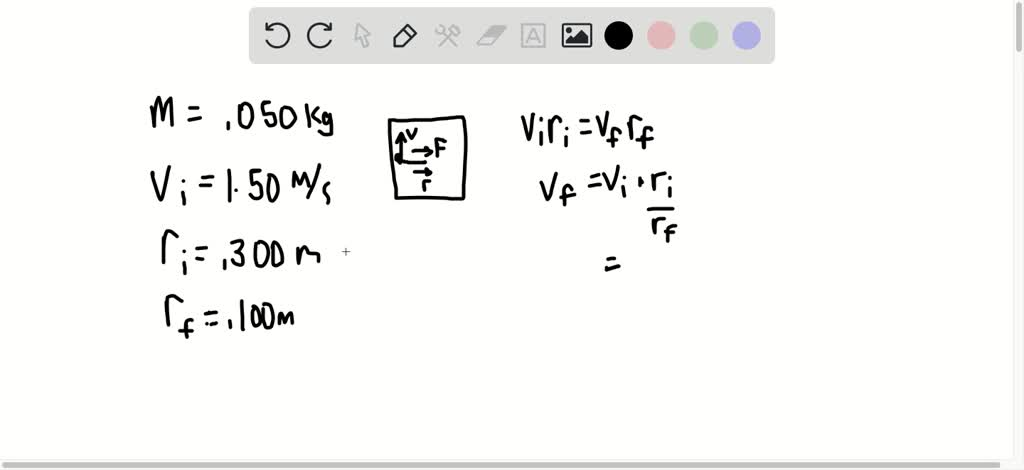1

# A puck of mass $m=50.0 \mathrm{g}$ is attached to a taut cord passing through a small hole in a friction less, horizontal surface (Fig. P 11.52). The puck is initia...

## Question

###### A puck of mass $m=50.0 \mathrm{g}$ is attached to a taut cord passing through a small hole in a friction less, horizontal surface (Fig. P 11.52). The puck is initially orbiting with speed $v_{i}=1.50 \mathrm{m} / \mathrm{s}$ in a circle of radius $r_{i}=0.300 \mathrm{m} .$ The cord is then slowly pulled from below, decreasing the radius of the circle to $r=0.100 \mathrm{m} .$ (a) What is the puck's speed at the smaller radius? (b) Find the tension in the cord at the smaller radius. (c) How

A puck of mass $m=50.0 \mathrm{g}$ is attached to a taut cord passing through a small hole in a friction less, horizontal surface (Fig. P 11.52). The puck is initially orbiting with speed $v_{i}=1.50 \mathrm{m} / \mathrm{s}$ in a circle of radius $r_{i}=0.300 \mathrm{m} .$ The cord is then slowly pulled from below, decreasing the radius of the circle to $r=0.100 \mathrm{m} .$ (a) What is the puck's speed at the smaller radius? (b) Find the tension in the cord at the smaller radius. (c) How much work is done by the hand in pulling the cord so that the radius of the puck’s motion changes from 0.300 m to 0.100 m?